## Info

Publication number
JP4043552B2
JP4043552B2 JP18559597A JP18559597A JP4043552B2 JP 4043552 B2 JP4043552 B2 JP 4043552B2 JP 18559597 A JP18559597 A JP 18559597A JP 18559597 A JP18559597 A JP 18559597A JP 4043552 B2 JP4043552 B2 JP 4043552B2
Authority
JP
Japan
Prior art keywords
sequence
error
detector
sample
values
Prior art date
Legal status (The legal status is an assumption and is not a legal conclusion. Google has not performed a legal analysis and makes no representation as to the accuracy of the status listed.)
Expired - Fee Related
Application number
JP18559597A
Other languages
Japanese (ja)
Other versions
JPH1069727A (en
Inventor
アール． プローンド， ジュニア ウィリアム
ジー． ブリス ウィリアム
イー． リード デイビッド
シー． サンデル リサ
ティー． ベーレンズ リチャード
Original Assignee
ブロードコム・コーポレーシヨン
Priority date (The priority date is an assumption and is not a legal conclusion. Google has not performed a legal analysis and makes no representation as to the accuracy of the date listed.)
Filing date
Publication date
Priority to US08/681,578 priority Critical
Priority to US08/681,678 priority
Priority to US08/681,578 priority patent/US5802118A/en
Priority to US08/681,678 priority patent/US5771127A/en
Application filed by ブロードコム・コーポレーシヨン filed Critical ブロードコム・コーポレーシヨン
Publication of JPH1069727A publication Critical patent/JPH1069727A/en
Application granted granted Critical
Publication of JP4043552B2 publication Critical patent/JP4043552B2/en
Anticipated expiration legal-status Critical
Expired - Fee Related legal-status Critical Current

• 238000005070 sampling Methods 0 claims description 83
• 239000011159 matrix materials Substances 0 description 40
• 230000001360 synchronised Effects 0 description 28
• 238000000034 methods Methods 0 description 13
• 230000000694 effects Effects 0 description 11
• 238000000354 decomposition Methods 0 description 3
• 230000001934 delay Effects 0 description 2
• 238000004458 analytical methods Methods 0 description 1
• 230000001276 controlling effects Effects 0 description 1

## Images

•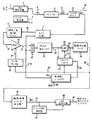•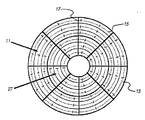•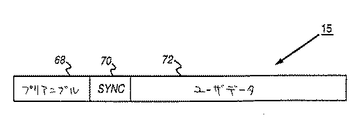•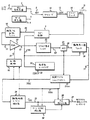•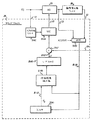•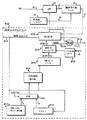•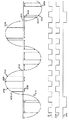•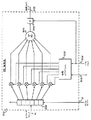•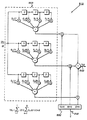•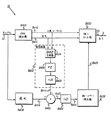•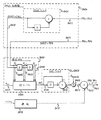•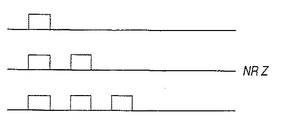•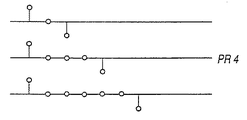•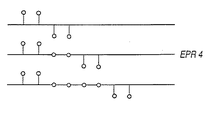•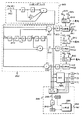••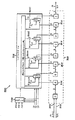•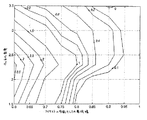•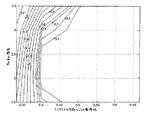•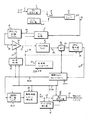•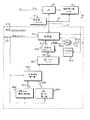•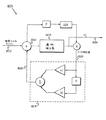•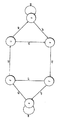••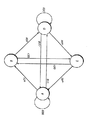•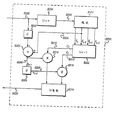## Classifications

• GPHYSICS
• G11INFORMATION STORAGE
• G11BINFORMATION STORAGE BASED ON RELATIVE MOVEMENT BETWEEN RECORD CARRIER AND TRANSDUCER
• G11B20/00Signal processing not specific to the method of recording or reproducing; Circuits therefor
• G11B20/10Digital recording or reproducing
• G11B20/10009Improvement or modification of read or write signals
• G11B20/10046Improvement or modification of read or write signals filtering or equalising, e.g. setting the tap weights of an FIR filter
• G11B20/10055Improvement or modification of read or write signals filtering or equalising, e.g. setting the tap weights of an FIR filter using partial response filtering when writing the signal to the medium or reading it therefrom
• GPHYSICS
• G11INFORMATION STORAGE
• G11BINFORMATION STORAGE BASED ON RELATIVE MOVEMENT BETWEEN RECORD CARRIER AND TRANSDUCER
• G11B20/00Signal processing not specific to the method of recording or reproducing; Circuits therefor
• G11B20/10Digital recording or reproducing
• G11B20/10009Improvement or modification of read or write signals
• GPHYSICS
• G11INFORMATION STORAGE
• G11BINFORMATION STORAGE BASED ON RELATIVE MOVEMENT BETWEEN RECORD CARRIER AND TRANSDUCER
• G11B20/00Signal processing not specific to the method of recording or reproducing; Circuits therefor
• G11B20/10Digital recording or reproducing
• G11B20/10009Improvement or modification of read or write signals
• G11B20/10037A/D conversion, D/A conversion, sampling, slicing and digital quantisation or adjusting parameters thereof
• GPHYSICS
• G11INFORMATION STORAGE
• G11BINFORMATION STORAGE BASED ON RELATIVE MOVEMENT BETWEEN RECORD CARRIER AND TRANSDUCER
• G11B20/00Signal processing not specific to the method of recording or reproducing; Circuits therefor
• G11B20/10Digital recording or reproducing
• G11B20/14Digital recording or reproducing using self-clocking codes
• G11B20/1403Digital recording or reproducing using self-clocking codes characterised by the use of two levels
• G11B20/1423Code representation depending on subsequent bits, e.g. delay modulation, double density code, Miller code
• G11B20/1426Code representation depending on subsequent bits, e.g. delay modulation, double density code, Miller code conversion to or from block codes or representations thereof

## Description


BACKGROUND OF THE INVENTION
The present invention relates to control of a storage system for a digital computer (for example, a magnetic disk drive, an optical disk drive, etc.). More specifically, the present invention relates to a sampled amplitude read channel using asynchronous subsampling of analog read signals, interpolated timing recovery, and remodulation / demodulation sequence detectors.

[Prior art]
Computer storage systems (eg, optical systems, magnetic systems, etc.) allow digital data by changing the surface characteristics of the disk onto the surface of a storage medium (typically in the form of a rotating magnetic disk or optical disk). Record. The digital data serves to modulate the operation of a light transducer (write head) that records binary data onto the disk in the form of a plurality of concentric or spiral tracks in the radial direction. For example, in a magnetic recording system, the digital data modulates the current in the write coil to record a series of magnetic flux transitions on the surface of a magnetizable disk. Also, for example, in an optical recording system, the digital data modulates the intensity of the laser beam to record a series of “pits” on the surface of the optical disc. When reading this recorded data, a read transducer (read head) positioned in the vicinity of the rotating disk detects changes on the medium and generates a corresponding sequence of pulses in the analog read signal. These pulses are then detected and decoded by a read channel circuit to reproduce the digital sequence.

Detection and decoding of pulses into a digital sequence has been done with a simple peak detector in conventional analog read channels. Also, more recent designs are done with discrete time sequence detectors in a sampled amplitude read channel. A discrete time sequence detector is preferred over a simple analog pulse detector. This is because the discrete time sequence detector can compensate for intersymbol interference (ISI) and is therefore less susceptible to channel noise. As a result, the discrete time sequence detector can increase the capacity of the storage system and increase its reliability. Known discrete time sequence detection methods include discrete time pulse detection (DPD), partial response (PR) using Viterbi detection, maximum likelihood sequence detection (MLSD), decision feedback equalization (DFE), and improved decision feedback. Examples include equalization (EDFE) and fixed delay tree search (FDTS / DF) using decision feedback.

In a conventional peak detection read channel, an analog circuit detects a peak in a continuous time analog read signal generated by a read head. This analog read signal is “segmented” into multiple bit cell periods and is interpreted between these time segments. When a peak exists within a certain bit cell period, it is detected as a “1” bit, while when there is no peak, it is detected as a “0” bit. The error is most likely to occur during detection when these bit cells do not match the analog pulse data correctly. Therefore, in order to minimize such a detection error, these bit cell periods are adjusted using timing recovery so that a peak occurs at the center of these bit cells if averaged. Since timing information is only available when a peak is detected, the input data stream is typically run length limited (RLL) to limit the number of consecutive “0” bits.

Even if a large number of pulses are closely packed on multiple data tracks to increase data density, intersymbol interference (ISI) or distortion caused in the read signal by pulses that are narrower and overlap each other, etc. Because of this, detection errors still occur. Based on this interference, the peak of the bit cell shifts from within the cell or the size of the peak decreases, so that a detection error eventually occurs. The impact of ISI is reduced by lowering the data density or by using an encoding scheme that ensures that the number of “0” bits between “1” and “1” bits is minimized. be able to. For example, a (d, k) run length limited (RLL) code constrains the minimum number of “0” bits between “1” and “1” bits to d, and consecutive “0” bits. Constrain the maximum number of to k. A typical (1,7) RLL 2/3 rate code encodes an 8-bit data word into a 12-bit codeword and satisfies the (1,7) constraint.

Sampled amplitude detection, such as partial response (PR) using Viterbi detection, can increase data density by compensating for the effects of intersymbol interference and channel noise. Unlike conventional peak detection systems, recording with sampled amplitude detects digital data by interpreting the actual value of the pulse data at discrete time points. For this purpose, the read channel comprises a sampling device that samples the analog read signal and a timing recovery circuit that synchronizes the sample with the baud rate (code bit rate). Before sampling these pulses, the variable gain amplifier adjusts the amplitude of the lead signal to the rated value, and a low-pass analog filter attenuates the channel noise and aliasing noise by filtering the lead signal. After sampling, a digital equalizer equalizes the sample values according to the desired partial response, and a discrete time sequence detector such as a Viterbi detector interprets these equalized sample values according to the context, The most likely sequence is determined for the digital data (ie, maximum likelihood sequence detection (MLSD) is performed). MLSD reduces the likelihood of detection errors by taking into account the effects of ISI and channel noise in the detection algorithm. This can increase the effective signal-to-noise ratio compared to traditional analog peak detection read channels, and achieve much higher data density for a given (d, k) constraint. can do.

Examples of applying sampled amplitude techniques to digital communication channels are already found in many documents. For example, Y. Kabal and S. Pasupathy's “Partial Response Signaling”, IEEE Trans. Commun. Tech., COM-23, 921-934, September 1975, Edward A. Lee and David G. See Messerschmitt's "Digital Communications", Kluwer Academic Publishers, Boston, 1990, and GD Forney, Jr.'s "Viterbi Algorithm", Proc. IEEE, Vol. 61, pp. 268-278, March 1973, etc. thing.

There are already many examples of applying sampled amplitude techniques to magnetic storage systems. For example, Roy D. Cideciyan, Francois Dolivo, Walter Hirt and Wolfgang Schott, “PRML System for Digital Magnetic Recording”, IEEE Journal on Selected Areas in Communications, Vol. 10, No. 1, January 1992, 38-56. Page, Wood et al., “Viterbi Detection of Class IV Partial Responses on Magnetic Recording Channels”, IEEE Trans. Commun., Vol. 34, No. 5, pp. 454-461, May 1986, by Coker et al. "PRML implementation in rigid disk drives", IEEE Trans. On Magnetics, Vol. 27, No. 6, November 1991, Carley et al., "Adaptive continuous time equalization after FDTS / DF sequence detection", Digest of The Magnetic Recording Conference, August 15-17, 1994, page C3, Moon et al., “Complexity-Limited Equalizer Design for Fixed Delay Tree Search Using Decision Feedback”, IEEE Trans. On Magnetics , Volume 30, Issue 5, September 1994, Abbott et al. Timing Recovery for Adaptive Decision Feedback Equalization of Storage Channels ", Globecom '90 IEEE Global Telecommunications Conference 1990, San Diego, CA, November 1990, pp. 1794-1799, Abbott et al.,“ Equalization and Off-track Interference "Performance of Digital Magnetic Recording Used", IEEE Transactions on Magnetics, Vol. 27, No. 1, January 1991, "Adaptive equalization in magnetic disk storage channel" by Cioffi et al., IEEE Communication Magazine, February 1990, See also Roger Wood's "Improved Decision Feedback Equalization", Intermag '90, etc.

The principles disclosed herein are applicable regardless of which discrete time sequence detection method is used. The present invention is applicable to all of the sequence detection methods listed above, as well as other detection methods not listed above, and even applicable to future developed technologies. It is.

Similar to the conventional peak detection system, timing recovery is necessary to accurately extract a digital sequence in sampled amplitude detection. Rather than performing continuous signal processing so that each peak coincides with the respective center of the bitcell period, as is done in a peak detection system, a sampled amplitude system uses multiple pulse samples at the baud rate. Synchronize. In conventional sampled amplitude read channels, timing recovery synchronizes the sampling clock by minimizing the error between the signal sample value and the estimated sample value. A pulse detector, or slicer, determines the sample value estimated from the read signal samples. These sample values can be estimated even in the presence of ISI, and these sample values can be sampled with analog signal sampling in a feedback system oriented by decision by using signal sample values together. It can also be used to synchronize.

Usually, by using a phase locked loop (PLL), it is possible to realize a feedback system whose direction is determined by determination using timing recovery. The PLL includes a phase detector that generates a phase error based on the difference between the estimated sample and the read signal sample. The PLL loop filter filters this phase error. The filtered phase error then acts to synchronize the channel samples with the baud rate.

Conventionally, the frequency of the sampling clock, which is typically the output of a variable frequency oscillator (VFO), is adjusted by this phase error. By controlling the sampling device, such as an analog-to-digital (A / D) converter, with the output of the VFO, the sampling can be synchronized to the baud rate.

Partial response (PR) using Viterbi detection is a method commonly used to detect digital data recorded from synchronous sample values in a sampled amplitude read channel, as described above. The most commonly used Viterbi-type sequence detection method is interleaving.WasA cost effective implementation that only requires two sliding threshold detectors, d = 0 rate 8 / 9PR4, and can improve bit error rate (BER) at high density, but more sophisticated There is d = 1 rate 2/3 EEPR4, which is an implementation that requires an add / compare / select (ASC) type sequence detector.

EEPROM 4The restriction of d = 1 in the read channel increases the minimum distance of the corresponding trellis code (and thus decreases the BER). In addition, this constraint reduces the complexity of the sequence detector by reducing the number of states, lowers its cost, and allows for a simpler configuration by establishing symmetry in the trellis model. To do. However, there are some disadvantages associated with the d = 1 system.

[Problems to be solved by the invention]
That is, in the d = 1 read channel, since the coding efficiency is reduced (compared with the rate 2/3 when d = 1 and the rate 8/9 when d = 0), the user data rate is also reduced. . Thus, in order to achieve higher user data rates, faster and more complex timing recovery and A / D circuits (ie higher frequency timing recovery VCOs and A / D converters) The data rate (code bit rate) must be increased. Such a configuration is not preferable. This is because it is not effective in terms of cost. In addition, this particular problem that occurs in the d = 1 read channel due to a decrease in the code rate also becomes a problem that can occur in the d = 0 read channel if the data rate is further increased. Furthermore, as described above, the d = 1 EPR4 / EEPR4 sequence detector has an extra cost to implement because the trellis model is more complex.

Thus, sampling for computer storage systems that can operate at high user data rates and densities without increasing the cost or further complexity of analog-to-digital converters, timing recovery VCOs or sequence detectors What is needed is an improved amplitude read channel. Another aspect of the invention is to use an encoding scheme that improves the performance of the d = 0 read channel with higher user data density when the analog read signal is subsampled.

The present invention has been made to solve the above-mentioned problems, and its object is to increase the cost of an analog / digital converter, a timing recovery VCO, or a sequence detector, or to make them more complicated. It is an object to provide a sampled amplitude read channel for a computer storage system that can operate at high user data rates and densities without. Another object is to provide an encoding scheme that improves the performance of the d = 0 read channel with higher user data density when the analog read signal is subsampled.

[Means for Solving the Problems]
A sampled amplitude for reading binary data from a sequence of interpolated discrete time sample values generated by sampling pulses in an analog read signal from a read head positioned on a disk storage medium according to the present invention A read channel is a sampled amplitude read channel in which the binary data is recorded at a predetermined baud rate, and (a) subsamples the analog read signal at a sampling rate of 9/10 or less of the baud rate. A sampling device that generates a subsampled value by: (b) an interpolator that generates an interpolated sample value in response to the subsampled value; and (c) the interpolated sample Discrete time detection that generates sequences detected from values And vessels, equipped with the above objects can be achieved.

In one embodiment, the discrete time detector operates according to code constraints that increase minimum distance error events.

In one embodiment, the code constraint is a (d, k) run-length limited code constraint (where d> 0).

In one embodiment, the interpolator (a) generates a frequency offset Δf that is proportional to the frequency difference between the sampling clock and the baud rate, and (b) modulo Ts and the frequency offset Δf. A modulo Ts accumulator that generates an interpolation interval τ by accumulating Ts, where Ts is a predetermined sample period of the sampling clock.

In one embodiment, the frequency offset generator (a) detects a phase error Δθ between the interpolated sample value and the estimated sample value; and (b) the phase error. A loop filter that generates the frequency offset Δf by filtering Δθ.

In one embodiment, the phase error detector comprises a discrete time pulse detector.

In one embodiment, the discrete time pulse detector comprises decision feedback equalization.

In some embodiments, the apparatus further comprises a first equalizer that generates a first equalized sample value and a second equalizer that generates a second equalized sample value. A sampled amplitude read channel, wherein: (a) the discrete time pulse detector is responsive to the first equalized sample value; (a) the discrete time pulse detector is the second Responds to the equalized sample value of.

In one embodiment, the interpolator further generates a data clock that synchronizes the clock of the discrete time detector.

In one embodiment, the discrete time detector operates according to a state transition table that is matched to a non-linear characteristic of the read signal.

In one embodiment, the non-linear effect is a partial erasure effect that is a non-linear reduction in the amplitude of the primary pulse caused by a secondary pulse located in the vicinity of the primary pulse.

In one embodiment, the discrete time detector (a) detects a preliminary sequence having one or more bit errors in response to the interpolated sample value; and (b) the preliminary sequence. A remodulator that converts to a sequence of estimated sample values; and (c) an adder that generates a sequence of sample error values in response to the interpolated sample values and the estimated sample values; (D) an error pattern detector that detects the magnitude and position of the bit error in the preliminary sequence in response to the sequence of sample error values; and (e) the magnitude and position of the bit error. And an error detector for responsively correcting the preliminary sequence.

In one embodiment, the remodulator comprises a partial erasure compensator that compensates for the nonlinear reduction of the amplitude of the primary pulse caused by a secondary pulse located in the vicinity of the primary pulse.

In one embodiment, the discrete time detector further comprises an error detection validity determiner that checks the validity of the detected error event.

A sampled amplitude read channel for reading binary data from a subsampled sequence of discrete time sample values generated by a subsampling pulse in an analog read signal from a read head positioned on a disk storage medium according to the present invention Is a sampled amplitude read channel in which the binary data is recorded at a predetermined baud rate, and (a) by subsampling the analog read signal at a sampling rate of 9/10 or less of the baud rate, A sampling device that generates a subsampled value; and (b) a timing recovery that generates a synchronized sample value by synchronizing the subsampled value; and (c) a binary from the synchronized sample value. Detect data, release Equipped with a time sequence detector, the object is achieved.

In one embodiment, the discrete time sequence detector operates according to a state transition table that is matched to a non-linear characteristic of the read signal.

In one embodiment, the non-linear effect is a partial erasure effect that is a non-linear reduction in the amplitude of the primary pulse caused by a secondary pulse located in the vicinity of the primary pulse.

In one embodiment, the sequence detector operates according to a state transition table substantially matched to the subsampled read signal.

In one embodiment, the sampling rate is within 10% of 1/2 of the baud rate.

In one embodiment, the state transition table is:

[Table 3]

It is.

In one embodiment, the state transition table is:

[Table 4]

It is.

In one embodiment, the timing recovery includes: (a) a slicer that generates an estimated sample value in response to the synchronization sample value; and (b) in response to a plurality of the estimated sample values. Control logic that generates at least one gradient factor; (c) an adder that generates a sample error value in response to the synchronization sample value and the estimated sample value; and (d) a sample error value. A multiplier for multiplying by the gradient factor, the multiplier having a multiplier used to generate an estimated phase error.

In one embodiment, (a) the timing recovery operates on a subsample value of an acquisition preamble recorded before user data, and (b) the acquisition preamble is longer than twice the baud rate. Written on the disk at periodic intervals.

A sampled amplitude read channel that reads binary data from a sequence of discrete time sample values generated by sampling pulses in an analog read signal from a read head positioned on a disk storage medium according to the present invention comprises: (A) a sampling device that generates a sequence of asynchronous sample values by sampling the analog read signal; and (b) an interpolator that generates synchronous sample values by interpolating the asynchronous sample values; (C) a discrete time detector for detecting the binary data from the synchronization sample value, and (a) detecting a binary sequence having one or more bit errors in response to the synchronization sample value; A demodulator, (b) converting the binary sequence into a sequence of estimated sample values A remodulator, (c) an adder that generates a sequence of sample error values in response to the synchronization sample value and the estimated sample value, and (d) responsive to the sequence of sample error value. An error pattern detector for detecting the magnitude and position of the bit error in the binary sequence; and (e) correcting the binary sequence in response to the magnitude and position of the bit error. A discrete-time detector having an error detector, whereby the above object is achieved.

In one embodiment, the demodulator outputs the code and magnitude of the binary sequence.

In one embodiment, the remodulator comprises an NRZI converter that converts the binary sequence into an NRZI sequence.

In one embodiment, the remodulator comprises a partial erasure compensator that compensates for the nonlinear reduction of the amplitude of the primary pulse caused by a secondary pulse located in the vicinity of the primary pulse.

In one embodiment, the error pattern detector comprises a plurality of discrete time filters matched to a predetermined error event.

In one embodiment, a sampled amplitude read channel further comprising means for converting the sequence of sample error values from a low order partial response region to a high order partial response region, wherein the error pattern detector comprises: The bit error is detected in the high-order partial response region.

In one embodiment, the demodulator comprises a PR4 sequence detector.

An embodiment further comprises an error detection validity determiner that validates the detected error event and enables the error corrector if the detected error event is valid. .

In one embodiment, the interpolator further generates a data clock that synchronizes the clock of the discrete time detector.

A sampled amplitude read channel that reads binary data from a sequence of discrete time sample values generated by sampling pulses in an analog read signal from a read head positioned on a disk storage medium according to the present invention comprises: (A) a sampling device that generates the sequence of discrete-time sample values by sampling the analog read signal; and (b) a timing that generates synchronized sample values by synchronizing the discrete-time sample values. Recovery, and (c) a discrete time detector for detecting the binary data from the synchronization sample value, and (a) a binary sequence having one or more bit errors in response to the synchronization sample value. A demodulator for detecting, (b) the estimated binary sequence A partial erasure compensator for converting into a sequence of signal values, compensating for the non-linear reduction of the amplitude of the primary pulse caused by a secondary pulse located in the vicinity of the primary pulse A remodulator, (c) an adder that generates a sequence of sample error values in response to the synchronization sample value and the estimated sample value, and (d) responsive to the sequence of sample error value. An error pattern detector for detecting the magnitude and position of the bit error in the binary sequence; and (e) correcting the binary sequence in response to the magnitude and position of the bit error. A discrete time detector with an error detector, whereby the above object is achieved.

An embodiment further comprises an error detection validity determiner that validates the detected error event and enables the error corrector if the detected error event is valid. .

A sampled amplitude read channel that reads binary data from a sequence of discrete time sample values generated by sampling pulses in an analog read signal from a read head positioned on a disk storage medium according to the present invention comprises: (A) a sampling device that generates the sequence of discrete-time sample values by sampling the analog read signal; and (b) a timing that generates synchronized sample values by synchronizing the discrete-time sample values. Recovery, and (c) a discrete time detector for detecting the binary data from the synchronization sample value, and (a) a binary sequence having one or more bit errors in response to the synchronization sample value. A demodulator for detecting, (b) the estimated binary sequence A remodulator that converts to a sequence of sample values; (c) an adder that generates a sequence of sample error values in response to the synchronization sample values and the estimated sample values; (d) the sample error values An error pattern detector for detecting the magnitude and position of the bit error in the binary sequence, each of which is matched to a predetermined error event, and a plurality of discrete time filters, An error pattern detector comprising: a peak detector for detecting a peak error event in response to a discrete time filter; and (e) in response to the magnitude and the position of the bit error, the 2 A discrete-time detector having an error corrector for correcting a binary sequence, whereby the above object is achieved.

A sampled amplitude read channel that reads binary data from a sequence of discrete time sample values generated by sampling pulses in an analog read signal from a read head positioned on a disk storage medium according to the present invention. (A) a sampling device that generates a sequence of discrete-time sample values by sampling the analog read signal; and (b) generates a synchronized sample value by synchronizing the discrete-time sample values. Timing recovery; and (c) a discrete time detector that detects the binary data from the synchronization sample value, and (a) a binary sequence having one or more bit errors in response to the synchronization sample value (B) the binary sequence estimated A remodulator that converts to a sequence of sample values; (c) an adder that generates a sequence of sample error values in response to the synchronization sample values and the estimated sample values; (d) the sample error values An error pattern detector that detects the magnitude and position of the bit error in the binary sequence in response to a sequence of: (e) an error detection validity determiner that validates the detected error event And (f) a discrete time detector having an error corrector that corrects the binary sequence in response to the magnitude and position of the bit error and the error detection validity determiner; With this, the above-described object is achieved.

The operation will be described below.

A sampled amplitude read channel for a computer disk storage system that records binary data is disclosed. This sampled amplitude read channel includes a sampling device that asynchronously samples pulses in an analog read signal from a read head located on a disk storage medium, an interpolated timing recovery that generates synchronous sample values, and a synchronization And a sequence detector for detecting binary data from the sample value. A sequence detector detects a bit error, a demodulator that detects a preliminary binary sequence that may have bit errors, a remodulator that remodulates to an estimated sample value, a means for generating a sample error value, and An error pattern detector, an error detection validity determination unit, and an error detector for correcting a bit error are provided. The remodulator comprises a partial erasure circuit that compensates for the non-linear reduction of the primary pulse amplitude caused by the secondary pulse located in the vicinity of the primary pulse. The error pattern detector includes a peak error pattern detector. The remodulator also includes means for disabling the error pattern detector if an error pattern is detected until the detected error pattern is completely processed. The error detection validity determiner checks the validity of the detected error event and, if it is valid, enables the error corrector operation.

By operating according to the d = 0 constraint, the demodulator can achieve higher user data rates while avoiding the increased cost and further complexity associated with the d = 1 read channel. The demodulator may also be implemented as a simple interleaved PR4 Viterbi detector that includes two sliding threshold detectors. Error pattern detectors and error correctors operate in higher partial response regions (eg, EPR4) to significantly improve sequence detector performance without increasing cost or complexity. be able to.

A subsampled discrete time read channel for a high data rate computer system is also disclosed. This read channel samples the analog read signal asynchronously at a rate significantly lower than the channel baud rate (code bit rate) and interpolates the asynchronous sample values to interpolate samples that are substantially synchronized to the baud rate. A value is generated and operates to detect digital data from the interpolated sample values. In order to improve the performance of the unconstrained read channel (d = 0 read channel) with the sub-sampling technique according to the present invention, which causes significant degradation at high data rates according to other methods than the present invention, The encoding scheme codes out data sequences that cause degradation.

Since the read signal is not sampled synchronously but is subsampled, it is not necessary to widen the A / D bandwidth in order to realize a higher user data rate. In addition, interpolated timing recovery eliminates the need for a synchronized VCO with a wide bandwidth. Actually, the VCO according to the present invention changes the frequency only when the read / write head transits between data zones on the disk (zone division recording is performed by dividing the disk into a predetermined number of zones and performing a predetermined operation on the disk. This is a technique in which a number of consecutive tracks are classified into one zone, and the data rate increases from the inner zone to the outer zone). In addition, the encoding scheme compensates for the performance degradation at high data rates due to sub-sampling by coding out a data sequence that causes degradation.

DETAILED DESCRIPTION OF THE INVENTION
This application is based on the following co-pending US patent application: US patent application Ser. No. 08 / 440,515 entitled “Sampled Amplitude Read Channel for Reading User Data and Embedded Servo Data from a Magnetic Medium”. No. 08 / 341,251, entitled “Sampled Amplitude Read Channel, Including Sample Estimation Equalization, Defect Scanning, Channel Quality, Digital Servo Demodulation, PID Filter for Timing Recovery, and DC Offset Control” US patent application Ser. No. 08 / 313,491 entitled “Improved Timing Recovery for Synchronous Partial Response Recording” and “Improved Defect-tolerant Sync Mark Detector for Sampled Amplitude Magnetic Recording” Related to US patent application Ser. No. 08 / 533,797. This application also describes several U.S. patents listed below: U.S. Pat. No. 5,359,631, entitled "Timing Recovery Circuit for Synchronous Waveform Sampling", "Viterbi-type sequence detector with reduced complexity". U.S. Pat.No. 5,291,499 entitled `` Methods and Apparatus of the Invention '', U.S. Pat.No. 5,297,184 entitled `` Gain Control Circuit for Synchronous Waveform Sampling '' And U.S. Pat. No. 5,424,881 entitled “Synchronous Read Channel”. All of the patent applications and patents listed above are assigned to the same assignee, and all of them are incorporated herein by reference.

These and other aspects and advantages of the present invention will become better understood when the following detailed description of the invention is read with reference to the accompanying drawings.

Referring now to FIG. 1, a detailed block diagram of a conventional sampled amplitude read channel is shown. During the write operation, user data 2 or preamble data (eg, 2T preamble data) from the data generator 4 is written on the medium. The RLL encoder 6 encodes the user data 2 into a binary sequence b (n) 8 according to the RLL constraint condition. The precoder 10 compensates the transfer function of the recording channel 18 and the equalizer filter and precodes the binary sequence b (n) 8 in order to create a precoded sequence ˜b (n) 12. The precoded sequence ~ b (n) 12 translates ~ b (N) = 0 to a (N) =-1 and ~ b (N) = 1 to a (N) = + 1 ( 14), the symbol a (n) 16 is converted. The write circuit 9 records the binary sequence on the medium by modulating the current in the recording head coil at the baud rate 1 / T in response to the symbol a (n) 16. The frequency synthesizer 52 supplies a baud rate write clock 54 to the write circuit 9. The frequency synthesizer 52 is adjusted by a channel data rate signal (CDR) 30 depending on which zone the recording head is located.

When reading a recorded binary sequence from the medium, the timing recovery 28 first locks to the write frequency by selecting the write clock 54 through the multiplexer 60 as an input to the read channel. Once locked to the write frequency, the multiplexer 60 selects the signal 19 from the readhead as an input to the read channel to obtain the acquisition preamble recorded on the disc prior to the recorded user data. . The variable gain amplifier 22 adjusts the amplitude of the analog read signal 58, and the analog filter 20 attenuates aliasing noise and at the same time performs initial equalization to obtain a desired response. The sampling device 24 samples the analog read signal 62 from the analog filter 20, and the discrete time equalizer filter 26 further equalizes the sample value 25 to obtain the desired response. In partial response recording, the desired response is often selected from Table 5, for example.

[Table 5]

After equalization, in order to adjust the amplitude of the read signal 58 and the frequency and phase of the sampling device 24 respectively, the equalized sample value 32 is converted into a gain control 50 that is oriented by decision and timing recovery. Circuit 28. Timing recovery adjusts the frequency of the sampling device 24 via line 23 to synchronize the equalized samples 32 to the baud rate. In order to align the timing recovery frequency to a predetermined value with respect to variations in temperature, voltage and process, the frequency synthesizer 52 provides a rough set value of the center frequency to the timing recovery circuit 28 via the line 64. Channel data rate (CDR) signal 30 adjusts the frequency range of synthesizer 52 according to the data rate for the current zone. The gain control 50 adjusts the gain of the variable gain amplifier 22 via the line 21 in order to match the magnitude of the frequency response of the channel with a desired partial response.

The equalized samples 32 are also sent to a discrete time sequence detector 34, such as a maximum likelihood (ML) Viterbi sequence detector. The discrete time sequence detector 34 detects a binary sequence {circumflex over (b)} (n) 33 estimated from the sample values. The RLL decoder 36 decodes the estimated binary sequence {circumflex over (b)} (n) 33 from the sequence detector 34 into the estimated user data 37. The data sync detector 66 detects a sync mark 70 (shown in FIG. 2B) in the data sector 15 in order to synchronize the operation of the RLL decoder 36 with the frame. If there is no error, the estimated binary sequence {circumflex over (b)} (n) 33 matches the recorded binary sequence b (n) 8, and the decoded user data 37 is recorded with the recorded user data 2. Match.

(data format)
FIG. 2A shows an example of a data format of a magnetic medium provided with a series of concentric data tracks 13. Here, each data track 13 has a plurality of sectors 15 in which servo wedges 17 are embedded. A servo controller (not shown) processes the servo data in the servo wedge 17, and positions the read / write head on a desired track in response to the servo data. Further, the servo controller processes servo bursts in the servo wedge 17 to keep the head positioned on the center line of a desired track while writing or reading data. Servo wedge 17 can be detected either by a simple discrete time pulse detector or by a discrete time sequence detector 34. If the sequence detector 34 detects servo data, the format of the servo wedge 17 will include a preamble and sync mark, similar to the user data sector 15.

FIG. 2B shows the format of the user data sector 15 including the acquisition preamble 68, the sync mark 70 and the user data 72. Timing recovery uses the acquisition preamble 68 to obtain the correct sampling frequency and phase before reading the user data 72, and the sync mark 70 delimits the beginning of the user data 72.

In order to increase the overall storage density, the disc is divided into an outer zone 11 with 14 data sectors per track and an inner zone 27 with 7 data sectors per track. In practical use, the disk is actually divided into a plurality of zones having different numbers of sectors per zone. In that case, data will be recorded in and detected from each zone at different data rates.

FIG. 3 illustrates an improved sampled amplitude read channel according to the present invention. In FIG. 3, the conventional sampled timing recovery 28 of FIG. 1 is replaced by an interpolated timing recovery B100. Further, the write frequency synthesizer 52 generates a baud rate write clock 54 that is applied to the write circuit 9 during a write operation, or is interpolated by the sampling device 24, the discrete time equalizer filter 26 during a read operation. Asynchronous read clock 54 that synchronizes the timing recovery B100 with the frequency (CDR30) for the current zone is generated. In an alternative embodiment, the first frequency synthesizer generates a write clock and the second frequency synthesizer generates a read clock.

Interpolated timing recovery B100 interpolates equalized sample values 32 to generate interpolated sample values B102 that are substantially synchronized with the current zone data rate. The discrete time sequence detector 34 detects an estimated binary sequence 33 representing user data from the interpolated sample values B102 (synchronized sample values). The interpolated timing recovery circuit B100 also generates a frequency synchronization data clock B104 that clocks the operations of the gain control 50, discrete time sequence detector 34, sync mark detector 66, and RLL decoder 36.

(Conventional timing recovery)
A general view of the conventional sampling timing recovery 28 shown in FIG. 1 is shown in FIG. 4A. The output 23 of the variable frequency oscillator (VFO) B164 controls the sampling clock of the sampling device 24, which is typically an analog to digital converter (A / D) in the digital read channel. Multiplexer B159 selects a non-equalized sample value 25 during acquisition, and selects an equalized sample value 32 during tracking, thereby avoiding the associated latency during the acquisition. Equalizer filter 26 is removed from the timing loop. The phase error detector B155 receives the sample value received via line B149 and the estimated sample value ~ Y from the sample value estimator B141, such as a slicer in a PR4 read channel with d = 0 on line B143.kIn response to the above, a phase error is generated. The loop filter B160 filters the phase error to generate a frequency offset Δf B167 that settles to a value proportional to the frequency difference between the sampling clock 23 and the baud rate. Frequency offset Δf B167, along with center frequency control signal 64 from frequency synthesizer 52, synchronizes sampling to the baud rate by adjusting sampling clock 23 at the output of VFO B164.

Zero phase start circuit B162 minimizes the initial phase error between sampling clock 23 and read signal 62 by stopping the operation of VFO B164 at the beginning of acquisition. This is done by disabling VFO B164, detecting a zero cross in analog read signal 62, and re-enabling VFO B 164 after a predetermined delay between the detected zero cross and the first baud rate sample.

(Interpolated timing recovery)
An interpolated timing recovery B100 according to the present invention is shown in FIG. 4B. The VFO B164 in the conventional timing recovery of FIG. 4A is replaced with a modulo Ts accumulator B120 and an interpolator B122. Further, the expected sample value generator B151 is the expected sample used by the phase error detector B155 in response to the interpolated sample value B102.Y k + τ To calculate the phase error during acquisition. Multiplexer B153 calculates the estimated sample value from slicer B141 to Yk + τIs made available by the phase error detector B155 during tracking. As will be described in detail later, data clock B104 is generated at the output of AND gate B126 in response to sampling clock 54 and mask signal B124 from modulo Ts accumulator B120. Phase error detector B155 and slicer B141 process the interpolated sample value B102 at the output of interpolator B122 instead of the channel sample value 32 at the output of discrete equalizer filter 26 of FIG. 4A. PID loop filter B161 controls a closed loop frequency response similar to loop filter B160 of FIG. 4A.

In the interpolated timing recovery according to the present invention, it is no longer necessary to lock the VFO to the reference frequency before acquiring the preamble, and the write clock 54 is multiplexed to the analog receive filter 20 (as in FIG. 1). That (60) is not necessary. Furthermore, the sampling device 24 and the discrete equalizer filter 26 are removed from the timing recovery loop, along with the delays associated with them. It is not necessary to multiplex around the equalizer filter 26 (B159) between acquisition and tracking. However, it is still necessary to acquire the preamble 68 before tracking the user data 72. For this purpose, similar to the zero phase start circuit B162 of FIG. 4A, at the beginning of acquisition, the zero phase start circuit B163 minimizes the initial phase error between the interpolated sample value and the baud rate. However, instead of stopping the operation of the sampling VFO B164, the interpolated timing recovery zero phase start circuit B163 calculates the initial phase error τ from the sample value 25 of the A / D 24, and this initial phase error is modulo Ts accumulator. Load to B120.

The modulo Ts accumulator B120, data clock B104, and interpolator B122 will be described in detail below.

(Interpolator)
The interpolator B122 of FIG. 4B can be understood with reference to FIG. 5, which shows a sampled 2T acquisition preamble signal B200. The target synchronization sample value B102 is shown as a black circle, and the asynchronous channel sample value 32 is shown as a vertical arrow. Below the sampled preamble signal is a timing chart representing the corresponding timing signals of sampling clock 54, data clock B104 and mask signal B124. As shown in FIG. 5, the preamble signal B200 is sampled slightly faster than the baud rate (target value rate).

The function of the interpolator is to estimate the target sample value by interpolating the channel sample values. For illustration purposes, consider linear interpolation, which is a simple estimation algorithm.

Y (N-1) = x (N-1) + [tau]. (X (N) -x (N-1)) (1)
Where x (N−1) and x (N) are channel samples around the target sample, and τ is proportional to the time difference between the channel sample value x (N−1) and the target sample value. This is the interpolation interval. The interpolation interval τ is generated at the output of the modulo Ts accumulator B120 that accumulates the frequency offset signal Δf B167 at the output of the PID loop filter B161.

τ = (ΣΔf) MOD Ts (2)
Here, Ts is the sampling period of the sampling clock 54. Since the sampling clock 54 samples the analog read signal 62 slightly faster than the baud rate, the data clock needs to be masked whenever the accumulated frequency offset Δf, ie the integer divided by Ts, increases by one. is there. The operation of the mask signal B124 generated by the data clock B104 and the modulo Ts accumulator B120 can be understood with reference to the timing chart of FIG.

If the interpolator implements the simple linear equation (1) described above, the channel sample values B202 and B204 are used to generate interpolated sample values corresponding to the target sample value B206. The interpolation interval τ B208 is generated according to equation (2) above. The next interpolated sample value corresponding to the next target value B210 is calculated from the channel sample values B204 and B212. This process “wraps around” and actually becomes τ B216 (ie, activates mask signal B124 by increasing the accumulated frequency offset Δf, ie the integer divided by Ts, by 1). Otherwise, the interpolation interval τ B214 is continued until it becomes larger than Ts. At this point, the data clock B104 is represented by the mask signal B124 so that the interpolated sample value corresponding to the target sample value B220 is calculated from the channel sample values B222 and B224 rather than from the channel sample values B218 and B222. Masked.

The simple linear interpolation of equation (1) only works when the analog read signal is sampled at a much higher frequency than the baud rate. Operating the channel at a higher frequency is undesirable because it increases its complexity and cost. Thus, in the preferred embodiment, interpolator B122 is implemented as a filter that calculates interpolated sample values in response to more than two channel samples.

An ideal discrete-time phase interpolation filter has a flat magnitude response and a constant group delay of τ.

Cτ(Ej ω) = Ej ωτ  (3)
This has an ideal impulse response.

sinc (π · (n−τ / Ts)) (4)
Unfortunately, (4) which is the above-mentioned non-causal infinite impulse response cannot be realized. Therefore, the impulse response of the interpolation filter is designed to be an approximation that best matches the ideal impulse response (4). This is obtained by minimizing the mean square error between the frequency response of the actual interpolation filter and the frequency response of (3) which is the ideal interpolation filter. This approximation can be improved by taking into account the spectrum of the input signal. That is, by minimizing the mean square error between the input spectrum multiplied by the actual interpolation spectrum and the input spectrum multiplied by the ideal interpolation spectrum, the following is obtained.

/ Cτ(Ej ω) X (ej ω-Cτ(Ej ω) X (ej ω(5)
Where / Cτ(Ej ω) Is the spectrum of the actual interpolation filter and x (ej ω) Is the spectrum of the input signal. “/” Indicates “bar”. From equation (5), the mean square error is expressed by the following equation (1).

[Expression 1]

Where x (ej ω) Is the spectrum of the read channel (for example, PR4, EPR4, EEPR4 and other partial response spectra in Table 5).

Actually, the above-described mean square error equation (1) can be rewritten as follows by specifying that the spectrum of the input signal is band-limited to a certain constant 0 ≦ ω ≦ απ. Here, 0 <α <1. That is
| X (ej ω) | = 0, but | ω | ≧ απ
It is. Then, Formula 1 becomes as follows.

[Expression 2]

As a solution to the minimization problem of Equation 2, for example, there is a method in which an actual interpolation filter is expressed by its coefficient, and a solution of a coefficient that can minimize the error from the viewpoint of the traditional mean square is obtained. .

An actual interpolation filter can be expressed as an FIR polynomial as shown in Equation 3 below.

[Equation 3]

Here, 2R is the number of taps in each interpolation filter, and the sample period Ts is normalized to 1. A mathematical differentiation method for an interpolation filter having an even number of coefficients will be described below. It would be possible within the skill of a person skilled in the art to modify the following algorithm so that an interpolation filter with an odd number of coefficients can be differentiated.

Substituting Equation 3 into Equation 2, the coefficient CτA desired mathematical formula can be obtained by (n).

[Expression 4]

The next step is the factor CτTaking the derivatives of equation 4 for (n) and initializing them to zero.

[Equation 5]

If carefully deformed, the following formula 6 is derived from the formula 5.

[Formula 6]

Where n0= -R, ..., 0,1, ..., R-1.

Define φ (r) as in Equation 7 below.

[Expression 7]

Further, when Expression 7 is substituted into Expression 6, the following Expression 8 is obtained.

[Equation 8]

Where n0= -R, ..., 0,1, ..., R-1.

Equation 8 is the coefficient Cτ(N) defines a set of 2R linear equations. Equation 8 can be expressed more simply in matrix form as follows:

ΦTCτ= Φτ
Where CτIs a column vector of the form

Cτ= [Cτ(-R), ..., cτ(0), ..., cτ(R-1)]t
ΦTIs a Toeplitz matrix of the form

[Equation 9]

ΦτIs a column vector of the form

Φτ= [Φ (-R + τ), ..., φ (τ), φ (1 + τ), ..., φ (R-1 + τ)]t  (6)
The solution of equation (6) is as in equation (7) below.

Cτ= ΦT -1Φτ  (7)
Where ΦT -1Is an inverse matrix whose solution can be obtained using a known method.

Table 6 shows 2R = 6, α = 0.8 and x (ej ω) = Coefficient C calculated from equation (7) when PR4τAn example of (n) is shown. FIG. 6 shows an embodiment of an FIR filter having six taps. Shift register B 250 receives channel samples 32 at sampling clock rate 54. Filter coefficient Cτ(N) is stored in the coefficient register file B252 and provided to the corresponding multiplier according to the current value of τB128. These coefficients are multiplied by the channel sample 32 stored in the shift register B250. The sum of the resulting products is taken (B254), and the sum is stored in the delay register B256. The coefficient register file B252 and the delay register B256 are clocked by the data clock B104 that realizes the above-described masking function.

[Table 6]

In an alternative embodiment not shown, a plurality of static FIRs having coefficients corresponding to different values of τ filter the sample values in shift register B250. Each filter outputs an interpolation value, and the current value τ B128 of the interpolation interval selects the output of the corresponding filter as the output B102 of the interpolator B122. In FIG. 6, since the coefficients of one filter are not updated at regular intervals, according to the embodiment using such a large number of filters, the speed of the interpolator B122 can be increased, and the entire read channel can be increased. Throughput can be increased.

(Interpolator with reduced cost)
In a more efficient and reduced cost embodiment, instead of storing all the coefficients of the interpolation filter in memory, the coefficient register file B252 shown in FIG.τ(N) is calculated in real time as a function of τ. Filter coefficient Cτ(N) can be calculated, for example, in real time according to a predetermined polynomial in τ (see, eg, US Pat. No. 4,866,647 entitled “Continuous Variable Digital Delay Circuit” assigned to Farrow) The disclosure of this patent is also incorporated herein by reference). In another preferred embodiment for calculating the filter coefficients in real time, the filter coefficients are estimated according to a matrix expression in which the ranks of these coefficients are lowered.

The bank of filter coefficients stored in the coefficient register file B252 is an M × N matrix AM × NCan be expressed as Where N is the depth of the interpolation filter (ie, the coefficient C in the impulse response calculated according to equation (7)).τ(Number of (n)), and M is the number of interpolation intervals (that is, the number of τ intervals). This more efficient and cost-effective embodiment isM × NRather than storing the entire matrix in memory, AM × NThis is realized by performing matrix factorization and singular value decomposition (SVD).

AM × NF matrixM × NMatrix and GN × NConsider the case where a matrix can be factored.

AM × N= FM × N・ GN × N
This replaces N with L (where L <N, more preferably L << N) and FM × NMatrix and GN × NBy reducing the size of the matrix, AM × NYou can make approximate values with lower ranks of matrices. In other words, when multiplied, the product is AM × NF closest to the matrixM × LMatrix and GL × NFind the matrix.

AM × N≒ FM × L・ GL × N
Next, as shown in FIG. 7, G as a bank of FIR filters B260 connected to receive channel sample values 32 (as will become more apparent upon reading the following description).L × NF as a lookup table B262 that realizes a matrix and uses τ B128 as an indexM × LBy realizing the matrix, the interpolation filter can be convolved. In an alternative embodiment, AM × NOne skilled in the art will appreciate that the matrix can be factored into three or more matrices (ie, A≈FGH...).

FM × LMatrix and GL × NThe preferred method for finding the matrix is to minimize the sum of the squared errors obtained by Equation 10 below.

[Expression 10]

The solution of Equation 10 is AM × NIt is obtained by singular value decomposition of the matrix. This singular value decomposition includes the following steps.

1. An SVD that can perform the following factorization (assuming that M ≧ N) is AM × NSteps to be performed on a matrix,
AM × N= UM × N・ DN × N・ VN × N,here
UM × NIs an M × N unitary matrix,
DN × NIs an N × N diagonal matrix {σ1, Σ2, ... σN} (Where σiIs AM × NSingular value of and σ1≧ σ2... ≧ σN≧ 0),
VN × NIs an N × N unitary matrix,
2. Diagonal matrix D reduced in size by selecting L maximum singular values σ, which is a predetermined numberL × LA step of generating

## EQU11 ##

3. UM × NExtracting the first L columns from the matrix reduced the size of UM × LForming a matrix;

[Expression 12]

4). VN × NExtracting the first L rows from the matrix reduced the size of VL × NForming a matrix;

[Formula 13]

5. FM × LMatrix and GL × NDefining the matrix to satisfy the following relationship:
FM × L・ GL × N= UM × L・ DL × L・ VL × N≒ AM × N
(For example, FM × L= UM × L・ DL × LAnd GL × N= VL × NAnd)
Is included.

As described above, in an embodiment with reduced cost using a polynomial and a reduced rank matrix, the interpolation filter coefficient Cτ(N) is calculated in real time as a function of τ. That is, the impulse response h (n) of the filter is approximated according to the following equation (14).

[Expression 14]

Here, f (i, τ) is a predetermined function in τ (for example, a polynomial in τ, that is, τ is the above FM × LL is an index of the matrix, and L is a degree that determines the accuracy of the approximation (for example, the degree of the polynomial or the above FM × LMatrix column width) and Gi(N) is a predetermined matrix (for example, a polynomial, that is, GL × NMatrix coefficient). As L increases, the approximate filter coefficient C of Equation 14τ(N) tends to approach the ideal coefficient obtained by equation (7). From Expression 14, the output of the interpolation filter output Y (x) is expressed as shown in Expression 15 below.

[Expression 15]

Where U (x) is the channel sample value 32 and N is the interpolation filter coefficient CτThe number of (n).

Referring again to FIG. 6, the coefficient register file stores the interpolation filter coefficient C according to Equation 14.τAfter calculating (n), these coefficients CτBy convolving (n) with the channel sample U (x) 32, an interpolated sample value B102 synchronized with the baud rate can be generated. However, by organizing Equation 15, a more efficient embodiment of the interpolation filter can be realized as shown in Equation 16 below.

[Expression 16]

FIG. 7 shows a preferred embodiment of the interpolation filter according to equation 16. In embodiments using polynomials, the function of τ is a polynomial in τ and the matrix Gi(N) is a coefficient of this polynomial. Further, in the embodiment using the lowered rank matrix, the function of τ is the above FM × LIt becomes an index of the matrix B262, and the second sum in Equation 16 is

[Expression 17]

As shown in FIG. 7, it is realized as a bank of FIR filters B260. In Equation 16, L is the depth of the approximate function f (i, τ) (for example, the degree of the polynomial, that is, FM × LN is the depth of the impulse response of the interpolation filter (that is, the number of coefficients in the impulse response). It has been found that the best performance / cost balance is obtained when N = 8 and L = 3. However, as IC technology advances and the cost per gate goes down, these values can be increased.

(D = 0 remodulation / demodulation detector)
In a sampled amplitude storage system using the d = 0 RLL constraint, the read channel is typically equalized to a PR4 response and the discrete time sequence detector is typically implemented as a pair of interleaved sliding threshold Viterbi detectors. The PR4 equalization is preferred because higher order d = 0 sequence detectors (eg, EPR4 and EEPR4) increase the number of states in the trellis model, making it more complex and more expensive to implement. Because it becomes. However, it is also possible to improve the conventional PR4 sequence detector by searching for the minimum distance error event in the EPR4 region and then correcting the output of the PR4 detector when an error event is detected. In this way, the performance of the sequence detector is not as complicated as d = 0 EPR4 and can be brought closer to the performance of the EPR4 detector at a lower cost than d = 0 EPR4.

A preferred embodiment of the improved PR4 detector used for the d = 0 read channel is shown in FIG. 8A. This detector operates according to the following steps.

1. Remodulate the output of a conventional PR4 sequence detector into a sequence of ideal PR4 sample values;
2. Generating a sequence of PR4 sample error values by subtracting the ideal PR4 sample value from the read signal sample value;
3. Converting PR4 sample error values to EPR4 sample error values;
4). Filtering EPR4 sample error values with a bank of filters matched to the dominant EPR4 error event;
5. The largest magnitude matched filter output is selected, and if the magnitude exceeds a predetermined threshold, if the correction is valid, the detected binary sequence of PR4 is corrected according to the decision ( That is, as a result, a valid PR4 sequence is obtained).

The improved PR4 detector of FIG. 8A demodulates the read signal by remodulating the detected binary sequence to an estimated PR4 sample sequence and then correcting the detected errors in the EPR4 domain. This detector will be referred to as a remod / demod detector.

Referring now to FIG. 8A in detail, the conventional PR4 sequence detector B400 detects the preliminary binary sequence B412 from the read signal sample value 32. The PR4 detector B400 is preferably implemented as a pair of interleaved sliding threshold Viterbi detectors. However, the transition code B410 in both interleaves is stored and used by a remodulator B402. The sign bit B410 is associated with the “1” bit and “0” bit output by the PR4 detector B400 in each interleave. For example, if a positive transition is detected in an even number of interleaves, the PR4 detector B400 outputs “+1” followed by a plurality of “+0” values until a negative transition is detected. The sign bit B410, along with the detected binary sequence B412, is used to remodulate to the ideal PR4 sample sequence B414.

The remodulator includes a signed PR4 / SNRZI converter B404 (SNRZI is a shortened form of signed NRZI), a partial erasure compensator B406, and a 1 + D filter B408. Details of these will be described later. The remodulated sample sequence B414 is subtracted from the current read signal sample B416 to generate a PR4 sample error sequence B420 (delay B418 handles the delays of PR4 sequence detector B400 and remodulator B402) In order to delay the read signal sample). The PR4 sample error sequence B420 is then passed through a 1 + D filter B422 to generate an EPR4 sample error sequence B424.

An error pattern detector B426 matched to the dominant EPR4 error event processes the EPR4 sample error sequence B424. If an EPR4 error event is detected, a corresponding correction signal B428 is provided to the error correction circuit B430. The error correction circuit B430 corrects error bits in the detected binary sequence B412 output by the PR4 sequence detector B400.

Details of the remodulator circuit B402 are shown in FIG. 8B. The remodulator circuit B402 includes an SNRZI converter B404, a partial erasure compensator B406, a 1 + D filter B408, a gain B430, and an adder B432. SNRZI converter B404 receives detected binary bits B412 (ie, 0 or 1) and associated sign bits (ie, ± 1 or ± 0) B410 from PR4 sequence detector B400. The 1/1 + D filter B 434 generates a corresponding SNRZI magnitude sample sequence B 436 by filtering the detected binary sequence B 412. The detected sign bit B410 of the binary sequence B412 is directly converted to an SNRZI code bit.

Alternatively, the SNRZI converter B404 may be implemented as a look-up table indexed by the detected binary sequence B412 and the associated code bit B410. In the look-up table embodiment, error propagation can be avoided even in the case of pseudo catastrophic error events (ie, errors that result in unmerged paths in the PR4 sequence detector B400). Entries in the lookup table are shown in Table 7.

[Table 7]

The detected binary sequence is an SNRZI sequence (SnThe partial erasure compensator B406 compensates for the non-linear reduction introduced in the pulse amplitude due to adjacent flux transitions by adjusting the size of the SNRZI sample. That is, Sn-1The size of the SNRZI sample in B438 isnB442 or Sn-2± A if there is a transition adjacent to any of B444PEB440 (where | ± APE| <1). Sn-1The size of B438 is SnB442 and Sn-2If there are transitions adjacent to both of B444, ± (APE× APE). In order to implement the partial erasure compensator B406, Sn, Sn-1And Sn-2The SNRZI sample represented as is S according to the entries shown in Table 8.n-1Modified value of (SPn-1Index of the look-up table B446 that outputs (represented as B448).

[Table 8]

Modified SNRZI sample SP after compensating for the effect of partial erasuren-1B448 is passed through a 1 + D filter B408 to convert the SNRZI samples into an estimated PR4 sample sequence B450. Since the AGC 50 loop attempts to compensate for the non-linear effects of partial erasure by adjusting the read signal amplitude to average PR4 magnitude, the gain multiplier B430 is remodulated. The adjustment made by the AGC 50 is compensated by adjusting the size of the PR4 sequence B450. The estimated PR4 sample sequence B413 at the output of the gain multiplier B430 is then subtracted from the actual read signal sample value B416 in the adder B432 to generate a PR4 sample error sequence B420.

Referring again to FIG. 8A, the PR4 sample error sequence B420 is passed through the 1 + D filter B422 to generate the EPR4 sample error sequence B424. The EPR4 sample error sequence B424 is then processed by an error pattern detector B426 that is matched to the minimum distance EPR4 error event. Examples of minimum distance EPR4 error events are shown in FIGS. 8C-8E. FIG. 8C shows the three minimum distance error events of the PR4 detector in the NRZ region, FIG. 8D shows the same error event in the PR4 region, and FIG. 8E shows the error event in the EPR4 region. . Note that the EPR4 error sequence of FIG. 8E can be generated by passing the corresponding PR4 error sequence of FIG. 8D through a 1 + D filter. Thus, the error pattern detector B426 can be implemented as a 1 + D filter followed by a bank of filters matched to the PR4 error sequence. To further simplify the circuit, the 1 + D filter B422 of FIG. 8A for converting to an EPR4 sample error sequence is combined with the 1 + D filter in error pattern detector B426, as shown in FIG. Single 1 + 2D + D2A filter B450 may be formed.

1 + 2D + D shown in FIG. 8F2The output of filter B450 is connected to filter bank B452. Each of these filters has an impulse response matched to a corresponding one of the PR4 error sequences shown in FIG. 8D. The output X1 B454 of the adder B456 corresponds to the first error sequence of FIG. 8D, the output X2 B458 corresponds to the second error sequence of FIG. 8D, and the output X3 B460 corresponds to the output of FIG. This corresponds to the third error sequence. The output of the matched filter is compared with a predetermined threshold, and if that threshold is exceeded, an error detection signal is asserted. For example, the comparator B462 sets the X1 output B454 to the threshold value (TH1AOr TH1B) And if X1 B454 exceeds its threshold, the F1 signal B464 is asserted. The comparator B462 also outputs a sign bit F11 B465 indicating the polarity of the detected error (ie, the error polarity shown in FIGS. 8C to 8E may be inverted).

If an error event associated with a dibit, quad bit or 6 bit transition sequence occurs, the threshold level compared to the output of the corresponding matched filter is reduced to compensate for the effect of partial erasure (here But partial erasure is a reduction in pulse amplitude caused by adjacent (one or more) pulses). For example, multiplexer B 470 may have Dn + 5If a dibit sequence is detected or erroneously detected (ie, erroneously detected) at B476 (ie, time Dn + 5And Dn + 4Threshold value TH1 if the NRZI bits at both are non-zero or both are zero)ASelect B472 to compare with X1 B454. Otherwise, the multiplexer B470 uses the threshold value TH1.BIs selected for comparison with X1 B454. Similarly, mux B478 is used if Dn + 5If a quad bit sequence was detected or misdetected (ie, detected in error) at B476 (ie, time Dn + 5, Dn + 4, Dn + 3And Dn + 2Threshold value TH2 if the NRZI bits in are all non-zero or all zero)AIs selected for comparison with X2 B458. Otherwise, the multiplexer B478 sets the threshold value TH2BIs selected for comparison with X2 B458. Finally, multiplexer B 480 is connected if Dn + 5If a 6-bit transition sequence is detected or erroneously detected (ie, detected in error) in B476 (ie, time Dn + 5, Dn + 4, Dn + 3, Dn + 2, Dn + 1And DnThreshold value TH3 if the NRZI bits in are all non-zero or all zero)AIs selected for comparison with X3 B460. Otherwise, the multiplexer B480 is the threshold TH3BIs selected for comparison with X3 B460. Threshold THXAIs the threshold THXBFIG. 8B shows the partial erasure reduction coefficient A.PECalculated as a value multiplied by B440. This circuit is also TH1A= TH2A= TH3AAnd TH1B= TH2B= TH3BIt can be simplified by setting to.

The MAX circuit B482 compares the matched filter outputs X1, X2 and X3 and asserts the signal FA, FB or FC corresponding to the matched filter output having the maximum absolute amplitude. Signals FA, FB and FC are used to correct the detected binary sequence, as will be described later.

The peak error detector circuit B484 is responsive to the matched filter outputs X1, X2 and X3 and the maximum absolute amplitude signals FA, FB and FC to provide the maximum matched filter output at time n B490 and the maximum matched filter at time n-1 B492. Compare with the output (B486). If the maximum filter output at time n is less than the output at time n-1, signal FAH B494 is asserted to indicate that a peak error signal has been detected. FAH signal B494 enables the operation of error correction circuit B430 in FIG. 8A.

In an alternative embodiment not shown, the signals FA, FB, FC and FAH in FIG. 8F are generated using the difference between the filter output and the comparator threshold rather than the filter output itself. The That is, the MAX circuit B482 outputs the filter output XkAnd the corresponding threshold THkCompare the three values calculated as the difference between and the peak error detector B484 finds the peak in these difference values. This embodiment is preferred if a different threshold is used for each error event.

Details of the error corrector circuit B430 of FIG. 8A are shown in FIGS. 8G and 8H. In FIG. 8G, F1-F3, FA-FC and FAH signal B428 from error pattern detector B426 are input to respective error event AND gates B496A-B496C. The outputs of these gates are enabled through AND gates B498A-B498C by INHIBIT signal B500. If the corresponding matched filter output exceeds the TH threshold (F1, F2 or F3), it is the largest error event (FA, FB or FC), and it is a peak error event ( If FAH B494 is asserted) and INHIBIT signal B500 is not asserted, an error event (C1, C2 or C3) is detected. The detected error event (C1, C2 and C3), error event code (F11, F22 and F33), detected binary sequence B412 and code bit B410 are error validator and corrector B502. Is input. Error validity determiner and corrector B502 corrects the detected binary sequence B412 (and sign bit 410) if the detected error event is valid.

The INHIBIT signal B500 operates as follows. That is, if a valid error event is detected, the INHIBIT signal B500 is asserted for a number of clock cycles equal to the length of the detected error event. That is, subsequent error events are not processed until the current error event is corrected.

The INHIBIT signal B500 is implemented using a counter B522, a register B524, a multiplexer B526, and an OR gate B504. If a valid error event is detected (B508A, B508B or B508C is asserted), the output of OR gate B504 sets the output of register B524 (ie, INHIBIT signal B500) high. Thereby, the AND gates B498A to B498C are disabled. The detected error event selects count value 3, 5 or 7 via multiplexer B526, respectively, and the output of OR gate B504 loads the count value into counter B522. Next, DATA CLOCK synchronizes the clock of counter B522. When this count reaches the final count, the TC signal B528 resets the register B524, thereby re-enabling the AND gates B498A to B498C.

The outputs of AND gates B498A-B498C, represented as C1, C2, and C3, respectively, correspond to three error events that can be detected in error pattern detector B426 by a matched filter. These signals are used to correct the detected binary sequence B412 (and sign bit B410) when shifting through a series of registers B520 as shown in FIG. 8H. However, before correcting the binary sequence according to the detected error event, the correctness of the correction itself is checked.

If, for example, the noise that causes the detection error has the same polarity as the read signal, the error event may be detected incorrectly. To clarify this point, consider the first error event shown in FIG. 8D. Assuming that the PR4 signal is erroneously detected as a dibit data sequence due to the influence of noise, correction for canceling this noise is performed on the detected PR4 sequence (+1, +0, −1) with a sequence (−1). , -0, +1). However, if the dibit data sequence is actually recorded at the same position as the added noise, a reverse polarity error event will be detected, and the correction at that time will be the sequence (+1 , +0, −1) is added to the detected sequence (+1, +0, −1). As a result, the corrected sequence is (+2, +0, -2). In this case, since the PR4 sequence detector B400 has made the correct determination, the detected binary sequence B412 should not be corrected.

Referring again to FIG. 8H, a circuit is provided that checks the validity of the detected error event before correcting the detected data sequence. Lookup table B528 evaluates detected error events against detected PR4 sequences. Only when a valid PR4 sequence is obtained by the correction, the detected binary sequence B412 (and the sign bit B410) output by the PR4 sequence detector B400 is corrected. That is, for each error event, the detected PR4 sequence must match the expected possible PR4 sequence. Otherwise, no correction will be made.

In operation, the look-up table B528 includes the detected error event (C1, C2 or C3), the sign of the error event (F11, F22 or F33 selected by the detected error event through multiplexer B530) and the corresponding detection. PR4 data (binary sequence B412 and sign bit B410) Dn + 6B506, Dn + 4B510, Dn + 2B514 and DnReceive at B518. If a C1 error event is detected, using Table 9 below, look-up table B528 is Dn + 6B506 and Dn + 4In B510, the detected PR4 sequence is compared with the expected PR4 sequence. If a match is found, Dn + 6And Dn + 4The corrected PR4 data in is inserted into the shift register B520. Otherwise, the detected PR4 sequence is stored again in shift register B 520 uncorrected. Similarly, if a C2 error event is detected, using Table 10 below, look-up table B528 is Dn + 6B506, Dn + 4B510, and Dn + 2At B514, the detected PR4 sequence is compared with the expected PR4 sequence. If a match is found, Dn + 6, Dn + 4And Dn + 2The corrected PR4 data in is inserted into the shift register B520. Otherwise, the detected PR4 sequence is stored again in shift register B 520 uncorrected. Finally, if a C3 error event is detected, using Table 11 below, lookup table B528 isn + 6B506, Dn + 4B510, Dn + 2B514 and DnAt B518, the detected PR4 sequence is compared with the expected PR4 sequence. If a match is found, Dn + 6, Dn + 4, Dn + 2And DnThe correct PR4 data at is inserted into shift register B520. Otherwise, the detected PR4 sequence is stored again in shift register B 520 uncorrected. The corrected binary sequence B412 (and sign bit B410) is then shifted out of the shift register B520 and further processed by the read channel.

[Table 9]

[Table 10]

[Table 11]

In the above discussion, the interpolated timing recovery of FIG. 4B has been described as an oversampled system. That is, after the analog read signal 62 is slightly oversampled (24), it is “downsampled” (ie, interpolated B122) to generate a sample value B102 that is synchronized to the baud rate. However, it is also possible to generate synchronous samples by “up-sampling” after the analog read signal has been significantly undersampled. This is done by sampling (24) at a rate much lower than the baud rate (eg, 2/3 of the baud rate) and then interpolating to the sample value of the baud rate using the interpolated timing recovery circuit of FIG. 4B ( B122). By performing sub-sampling and interpolation, the A / D speed constraint is relaxed, and the timing recovery VCO B 164 of FIG. 4A becomes unnecessary. As a result, the overall throughput of the read channel can be improved.

In order to determine the extent to which subsampling degrades the performance of the read channel, i.e. to find a threshold where the performance degradation is more significant than the gain of user data rate obtained by subsampling the read signal. Computer simulation was performed. First, the subsampled read channel was simulated without imposing any sign constraints (d = 0). The result is shown in FIG. 9A. This graph shows contours of distance loss (performance degradation) caused by subsampling (reducing bandwidth as a fraction of baud rate) for various user data densities (number of user data bits per linear track inch). Plot. As shown in FIG. 9A, a read channel operating at a subsampled rate without a sign constraint (d = 0) can rapidly perform at higher data densities as the amount of subsampling increases. descend.

The same computer simulation was performed after introducing a code constraint that increased the minimum distance error event for the maximum likelihood sequence detector. Specifically, the RLL d = 1 constraint was introduced. The result is shown in FIG. 9B. As shown in FIG. 9B, the code constraint greatly improves the performance of the read channel even at high user data density. In fact, there is virtually no performance degradation for sub-sampling up to half the baud rate and user density of 3.5. One skilled in the art will recognize that other similar code constraints other than d = 1 may also improve performance in the presence of subsampling.

A filter matched to the pulse shape p (t) for both the uncoded (d = 0) and d = 1 code constraints cases, and xkA simulation was performed for an optimal MLSD sequence detector, including a sampler that generates = x (kT), a noise whitening filter, and a Viterbi (or equivalent) detection algorithm. Thus, a result similar to FIGS. 9A and 9B is a theoretical limit to the best possible performance that changes the degree of sub-sampling. Practical sequence detection methods are approximations of optimal MLSD, and they also degrade as the degree of subsampling increases (as bandwidth decreases).

(Conventional d = 1 subsampled read channel)
Referring back to FIG. 9B, when using the code constraint of d = 1 and subsampling at a rate up to approximately half the baud rate, there is virtually no degradation in the performance of the optimal MLSD read channel. This is good news. This is because more practical read channels will also operate at similar subsampling rates without significant performance degradation. Sub-sampling was performed at 2/3 of the baud rate, and a computer simulation was performed for the conventional d = 1, rate 2/3 read channel using the above-described interpolated timing recovery. It was confirmed that it corresponds to.

A preferred embodiment according to the invention of a d = 1 subsampled read channel is shown in FIG. This embodiment is similar in form and function to the read channel shown in FIG. However, since this embodiment is a d = 1 system, the precoder 10 of FIG. 3 is not necessary. Also, the cutoff frequency of the analog receive filter 20 is lowered to attenuate the increased aliasing noise caused by subsampling the lead signal. In the interpolated timing recovery B100, as shown in FIG. 11, a DFE transition detector B274 is used instead of the slicer of FIG. 4B. Also, the first equalizer 26 implements EPR4 equalization optimized for the timing recovery transition detector B274, and the second equalizer B270 performs the d = 1 sequence detector 34. Optimized EEPR4 equalization is realized.

Referring now to FIG. 11, the phase error detector B272 detects the interpolated sample value B102 and the transition P expected between acquisitions.kB288 or transition detected during tracking ~ PkA phase error estimate is calculated as a function of any of B284. The expected transition generator B286 of FIG. 11 operates similarly to the generator of FIG. 4B. That is, this generator B286 uses the transition P predicted by using the state machine.kB288 is generated, thereby calculating the phase error estimate while acquiring the acquisition preamble.

FIG. 12 is a block diagram of the DFE transition detector B274 of FIG. The decision feedback equalization (DFE) of FIG. 12 filters the data estimate B281 calculated as the sign of the sample value at the estimated transition at the output of the transition detector B276 (B278) and the output of the filter at the adder B282. This is done by adding B280 to the interpolated sample B102. As a result, the equalized orientation determined by the decision improves the accuracy of the transition detector B 276 when the analog read signal 62 is subsampled.

For d = 1 read channel, the sequence detector 34 shown in FIG. 10 can be implemented using conventional techniques. Preferably, it is implemented as a complex reduced Viterbi-type sequence detector. Referring again to FIG. 9B, sub-sampling at a rate up to half the baud rate does not affect the optimal MLSD system. The conventional read channel is also in the presence of sub-sampling. It shows that it works properly. As a result of computer simulation, when a conventional code with d = 1 and rate 2/3 is used, a preferable sub-sampling rate is determined to be 2/3 of the baud rate. In practical use, a sampling rate slightly higher than 2/3 of the baud rate (e.g., 1% to 5% higher) is used to perform the masking operation of the data clock B104 as already described with reference to FIG. Selected. Therefore, in FIG. 11, the frequency synthesizer 52 synchronizes the clocks of the A / D 24, the discrete time equalizer filter 26 and the interpolator B122 at a rate slightly exceeding 2/3 of the baud rate. Also, the frequency synthesizer 52 synchronizes the clock of the data clock AND gate B126 at a rate slightly exceeding the baud rate (ie, the output 54 of the frequency synthesizer 52 is increased by 1/3 (B121) and then the AND gate B126. Used to synchronize clocks).

Instead of a conventional Viterbi-type sequence detector and interpolated timing recovery, an improved sequence detector matched to the subsampled trellis model is used. Although a sequence detector matched to a subsampled read signal has reduced performance (its performance is closer to that of a conventional peak detector), its complexity is greatly reduced. In the next section, alternative embodiments according to the invention will be described.

(Matched d = 1 subsampled read channel)
The matched d = 1 subsampled sequence detector is defined as follows: That is, a detector that improves the state transition diagram of a conventional d = 1 sequence detector to match the state transitions of a subsampled sequence detector. FIG. 13A is a state transition diagram of a conventional full sample rate system with EPR4, d = 1. In this state diagram, each circle represents the state in the sampled lead signal (sample case), and the straight line with an arrow indicates the next state from the current state when the next input sample value is given. Represents a transition to Each state transition is labeled with the reference symbol s / b, where s represents the sample value and b represents the corresponding bit value of the detected binary sequence. .

A preferred embodiment according to the present invention for a matched d = 1 subsampled sequence detector is for subsampling at a rate half the baud rate and equalizing to an EPR4 response. The sub-sampling is performed using a conventional synchronous sampling PLL as shown in FIG. 4A (ie, sampling the read signal synchronously at half the baud rate). Alternatively, sub-sampling may be performed by asynchronously sampling the read signal at a rate slightly higher than 1/2 of the baud rate and then synchronizing to 1/2 of the baud rate through interpolation, thereby interpolating the timing recovery circuit of FIG. 4B. Can also be implemented.

An improved state transition diagram corresponding to 1/2 subsample rate, EPR4, d = 1 read signal is shown in FIG. 13B. Each state transition is labeled with a reference sign (s1, s2) / (b1, b2). Here, (s1, s2) represents two signal sample values, and (b1, b2) represents a 2-bit value corresponding to the detected binary sequence. As can be appreciated, the s1 and s2 sample values differ between state transitions that leave some particular state. Thus, sub-sampling at a rate half the baud rate is equivalent to evaluating only one of the two sample values, ie, only s1 or s2.

If only the s2 sample value is evaluated, the state transition diagram of FIG. 13B can be simplified by combining state C and state D and combining state A and state F. This is because these state transitions are equivalent. To clarify this point, consider the case where the current state is A. If the input sample value s2 is 1, the detected output bit is 10, and the next state is C. On transition from state C, if the next input sample value s2 is 0, the detected output bit is 00 and the next state is D. Similarly, if the next input sample value s2 is also 0, the detected output bit is again 00 and the next state remains D. On transition from state C, if the next input sample value s2 is -a, the detected output bit is 01 and the next state is E. Similarly, in the transition from state D, if the next input sample value s2 is -a, the detected output bit is 01 and the next state is E. Thus, states C and D can be combined. This is because an input sample sequence that ends in state E produces the same output bit sequence. A similar analysis will show that states A and F can be combined without degrading performance.

As a result of combining states C and D and states A and F, a simplified state transition diagram is shown in FIG. 13C. Since there are only four states as opposed to the six states in the conventional EPR4 diagram shown in FIG. 13A, the implementation of the ACS type Viterbi sequence detector has been greatly reduced.

However, for sub-sampling, the phase error detector in the timing recovery circuit must be modified (whether synchronous sampling in FIG. 4A or synchronous interpolation in FIG. 4B). Note that the simplified state transition diagram of FIG. 13C is similar to the conventional PR4 state diagram. In fact, if all of the channel transitions occur in an interleave (eg b2), the state diagram is exactly the same as the PR4 diagram. As a result of computer simulation, with some exceptions, a conventional PR4 read channel phase error detector may produce a valid phase error estimate for an EPR4 subsampled read channel according to the present invention. confirmed. For example, if the transition occurs from one interleave to the other, the estimated phase error is correct in magnitude but not in sign. This indicates that the timing loop can become unstable in the presence of random data.

A computer search was performed to determine a data sequence that could lead to an incorrect phase error estimate. This search was realized by placing a sampled read signal with a known timing offset into a conventional PR4 phase error detector and measuring the calculated phase error for all possible data sequences. Along with this, the algorithm for the phase error detector was subsequently modified to compensate for those data sequences that resulted in invalid phase error.

FIG. 14 is a block diagram of an improved PR4 phase error detector B290 used for a matched, d = 1, subsampled read channel according to the present invention. Again, instead of phase error detector B155 in the synchronously sampled timing recovery circuit of FIG. 4A or phase error detector B155 in the interpolated timing recovery circuit of FIG. 4B, the improved PR4 detector of FIG. Note that B290 can be used. In operation, the synchronous sample value B292 is input to a slicer (threshold detector) B294. The slicer B294 outputs the estimated ideal sample value B296 (in the case of EPR4, the estimated output value can take a value selected from −2, −1, 0, +2 and +2). The estimated sample value B296 is then delayed (B297), thereby delaying the estimated sample value S.n, Sn-1, Sn-2And Sn-3Generate the sequence. Sample error value en-1B298 is the read signal sample value B292 (delayed by one clock cycle (B302)) Sn-1Generated in adder B300 by subtracting from estimated sample value B304. Sample error value en-1B298 is delayed (B306) so that the second sample error value en-2B308 is generated. The sample error value is then multiplied by a coefficient C in each multiplier B314 and B316.0B310 and C1Scaled by B312. Where the scaling factor C0B310 and C1B312 is an estimated sample value S to compensate for a data sequence that may result in an incorrect phase error estimate.n, Sn-1, Sn-2And Sn-3Is calculated as a function of The logic B 322 is a sample value estimate S for generating a coefficient.n, Sn-1, Sn-2And Sn-3Contains two look-up tables indexed by. Here, the table entry was obtained according to the computer search described above. Coefficient C0The value of B310 is shown in Table 12 and the coefficient C1The value of B312 is shown in Table 13.

[Table 12]

[Table 13]

The scaled sample error signals at the outputs of multipliers B314 and B316 are added in adder B318. The output of the adder B318 is a phase error estimated value B320. This phase error estimated value is input to the timing loop filter of FIG. 4A or 4B as described above.

(Subsampled timing acquisition)
Referring back to FIG. 2B, each sector 15 of data includes an acquisition preamble field 68 that is used to synchronize timing recovery 28 to the baud rate before reading user data 72. The resulting read signal during acquisition is, for example, a periodic waveform such as the sinusoidal 2T acquisition preamble shown in FIG. As already described with reference to FIG. 4B, the periodicity of the preamble 68 facilitates the generation of expected sample values that are used to calculate the phase error. The reference symbol NT represents the period of the preamble when it is written on the disk (for example, when the symbol sequence a (n) 16 written on the disk is 101010101010101,... For a 2T preamble). .

Referring again to the conventional timing recovery circuit shown in FIG. 4A, the PLL processes the acquisition preamble 68 to synchronize the sampling VFO clock 23 to the baud rate. Also, for the interpolated timing recovery shown in FIG. 4B, the acquisition preamble 68 is processed to calculate a starting value for the interpolation interval τ B 128 before tracking the user data 72. In any case, the acquisition time should be as short as possible so that the length of preamble 68 can be minimized to allow more disk space for user data.

As a result of computer simulation, it has been found that the acquisition process can be optimized by lengthening the period of the acquisition preamble 68 in the presence of subsampling. In the case of the d = 1 read channel in FIG. 10, the 3T acquisition preamble (100100100...) Is preferable when subsampling at a rate 2/3 of the baud rate, and 4T when subsampling at a rate half the baud rate. The acquisition preamble (100010001000 ...) is preferred.

The objects of the present invention are fully realized by the various embodiments disclosed hereinabove. However, those skilled in the art will appreciate that various aspects of the present invention can be realized by other different embodiments without departing from the essential function of the present invention. For example, synchronous sampling timing recovery and interpolation may be used in combination by sampling at a half baud rate and then performing upsampling at a phase fixed to the full baud rate. it can. All such modifications and other modifications are within the scope of the invention. The specific embodiments disclosed above are for illustrative purposes only and are not intended to limit the scope of the present invention. The scope of the invention should be properly construed by the appended claims.

【The invention's effect】
According to the present invention, at least the following effects can be obtained. That is, sampling for computer storage systems that can operate at high user data rates and densities without increasing the cost or complicating them of analog-to-digital converters, timing recovery VCOs or sequence detectors Amplitude read channels can be provided.

Also, an encoding scheme can be provided that improves the performance of the d = 0 read channel with higher user data density when the analog read signal is subsampled.
[Brief description of the drawings]
FIG. 1 is a block diagram of a conventional sampled amplitude read channel in which timing recovery synchronizes sampling of an analog read signal to a baud rate.
FIG. 2A is a diagram showing an example of a data format of a magnetic disk having a plurality of concentric tracks composed of a plurality of user data sectors and a plurality of embedded servo data sectors.
FIG. 2B is a diagram showing an example of a format of a user data sector.
FIG. 3 is an improved sampled amplitude read channel according to the present invention having interpolated timing recovery to generate interpolated sample values and a synchronized data clock for clock alignment operation of a discrete time sequence detector. FIG.
FIG. 4A is a detailed block diagram of sampling timing recovery according to the prior art with a synchronized sampling VFO.
FIG. 4B is a detailed block diagram of interpolated timing recovery in accordance with the present invention with asynchronous sampling and an interpolator that generates interpolated sample values substantially synchronized to the baud rate.
FIG. 5 shows channel samples associated with interpolated baud rate samples when reading the acquisition preamble.
FIG. 6 is a diagram showing an embodiment of an FIR filter for a timing recovery interpolator.
FIG. 7 is a diagram illustrating an alternative embodiment for a timing recovery interpolator.
FIG. 8A is a general view of an improved PR4 sequence detector (remodulation / demodulation detector) used for the d = 0 read channel according to the present invention.
8B shows in detail the remodulator of the remodulation / demodulation sequence detector of FIG. 8A.
FIG. 8C illustrates a dominant error event in the NRZ region of a sampled amplitude read channel.
FIG. 8D illustrates a dominant error event in the PR4 region of a sampled amplitude read channel.
FIG. 8E illustrates a dominant error event in the EPR4 region of a sampled amplitude read channel.
8F shows in detail an error pattern detector with a bank of filters matched to the dominant error events of FIGS. 8C-8E.
FIG. 8G is a diagram showing a circuit for correcting an error included in a detected binary sequence output by a PR4 Viterbi detector when the error pattern detector of FIG. 8F detects an error.
FIG. 8H is a diagram showing a circuit for checking the validity of a detected error pattern.
FIG. 9A is a contour plot representing minimum distance loss as a function of the degree of subsampling and channel density used in a d = 0 read channel.
FIG. 9B is a contour plot representing minimum distance loss as a function of the degree of subsampling and channel density used in the d = 1 read channel.
FIG. 10 shows a preferred embodiment according to the present invention for a d = 1 subsampled read channel.
FIG. 11 is a block diagram illustrating interpolated timing recovery of a d = 1 subsampled read channel according to the present invention.
FIG. 12 is a diagram illustrating an embodiment of a DFE transition detector according to the present invention used for timing recovery of a d = 1 subsampled read channel.
FIG. 13A is a state transition diagram of a conventional EPR4 Viterbi type sequence detector.
FIG. 13B is a state transition diagram of an improved EPR4 Viterbi sequence detector matched to a read signal subsampled at half the baud rate.
13C is a simplified version of the state transition diagram shown in FIG. 13B.
FIG. 14 is a block diagram illustrating a phase error detector used in a d = 1, EPR4, subsampled read channel in accordance with the present invention.
[Explanation of symbols]
4 Data generator
6 RLL encoder
9 Write circuit
10 Precoder
18 Magnetic / optical recording channels
20 Analog filters
22 Variable gain amplifier
24 A / D converter
26 Discrete-time equalizer filter
34 Discrete-time sequence detector
36 RLL decoder
50 Gain control
52 frequency synthesizer
66 Data Sync Detector
B100 Interpolated timing recovery

## Claims (1)

1. In a sampled amplitude read channel that reads binary data from a sequence of interpolated discrete time sample values generated by sampling pulses in an analog read signal from a read head located on a disk storage medium A sampled amplitude read channel in which the binary data is recorded at a predetermined baud rate,
A sampling device that generates subsampled values by subsampling the analog read signal at a sampling rate of 9/10 or less of the baud rate;
An interpolator for generating interpolated sample values in response to the subsampled values;
A discrete time detector for generating a sequence detected from the interpolated sample values;
The discrete time detector comprises:
A demodulator for detecting a preliminary sequence having one or more bit errors in response to the interpolated sample value;
A remodulator that converts the preliminary sequence into a sequence of estimated sample values;
An adder that generates a sequence of sample error values in response to the interpolated sample values and the estimated sample values;
An error pattern detector for detecting the magnitude and position of the bit error in the preliminary sequence in response to the sequence of sample error values;
An error detector that corrects the preliminary sequence in response to the magnitude and position of the bit error;
A sampled amplitude read channel , wherein the remodulator comprises a partial erasure compensator that compensates for the non-linear reduction of the amplitude of the primary pulse caused by a secondary pulse located in the vicinity of the primary pulse .
JP18559597A 1996-07-29 1997-07-10 Sampled amplitude read channel Expired - Fee Related JP4043552B2 (en)

## Priority Applications (4)

Application Number Priority Date Filing Date Title
US08/681,578 1996-07-29
US08/681,678 1996-07-29
US08/681,578 US5802118A (en) 1996-07-29 1996-07-29 Sub-sampled discrete time read channel for computer storage systems
US08/681,678 US5771127A (en) 1996-07-29 1996-07-29 Sampled amplitude read channel employing interpolated timing recovery and a remod/demod sequence detector

## Publications (2)

Publication Number Publication Date
JPH1069727A JPH1069727A (en) 1998-03-10
JP4043552B2 true JP4043552B2 (en) 2008-02-06

# Family

## Family Applications (2)

Application Number Title Priority Date Filing Date
JP18559597A Expired - Fee Related JP4043552B2 (en) 1996-07-29 1997-07-10 Sampled amplitude read channel
JP2007053541A Pending JP2007179739A (en) 1996-07-29 2007-03-02 Sampled amplitude read channel

## Family Applications After (1)

Application Number Title Priority Date Filing Date
JP2007053541A Pending JP2007179739A (en) 1996-07-29 2007-03-02 Sampled amplitude read channel

## Country Status (2)

EP (1) EP0822552A3 (en)
JP (2) JP4043552B2 (en)

## Families Citing this family (7)

* Cited by examiner, † Cited by third party
Publication number Priority date Publication date Assignee Title
US6519715B1 (en) 1998-05-22 2003-02-11 Hitachi, Ltd. Signal processing apparatus and a data recording and reproducing apparatus including local memory processor
JP3950021B2 (en) * 2002-07-31 2007-07-25 株式会社東芝 Run length limited code generation method, run length limited code recording / reproducing apparatus, and run length limited code recording / reproducing method
CN1729528A (en) * 2002-10-23 2006-02-01 松下电器产业株式会社 Frequency and phase control apparatus and maximum likelihood decoder
JP4665597B2 (en) 2005-05-10 2011-04-06 ソニー株式会社 Phase synchronization apparatus and method, data reproduction apparatus and method, and program
US8270269B2 (en) 2005-07-07 2012-09-18 Panasonic Corporation Timing extraction device and video display device
WO2008102475A1 (en) * 2007-02-21 2008-08-28 Panasonic Corporation Maximum likelihood decoder and information reproducing device
JP5173021B2 (en) * 2008-05-19 2013-03-27 アギア システムズ インコーポレーテッド System and method for mitigating delay in a data detector feedback loop

## Family Cites Families (1)

* Cited by examiner, † Cited by third party
Publication number Priority date Publication date Assignee Title
US5576904A (en) * 1994-09-27 1996-11-19 Cirrus Logic, Inc. Timing gradient smoothing circuit in a synchronous read channel

## Also Published As

Publication number Publication date
EP0822552A2 (en) 1998-02-04
JPH1069727A (en) 1998-03-10
JP2007179739A (en) 2007-07-12
EP0822552A3 (en) 2000-04-05

## Similar Documents

Publication Publication Date Title
US5345342A (en) Disk drive using PRML synchronous sampling data detection and asynchronous detection of embedded sector servo
US4890299A (en) Fast timing acquisition for partial-response signalling
US7502427B2 (en) Asynchronous servo RRO detection employing interpolation
US8837068B1 (en) Two dimensional magnetic recording servo system adaptive combination
US5220466A (en) Method and apparatus for digital filter control in a partial-response maximum-likelihood disk drive system
US6201832B1 (en) Synchronous/asynchronous data detection apparatus for use in a magnetic recording/playback system
TWI227017B (en) Regeneration apparatus for the digital data
US6005727A (en) Servo decoder for decoding an error correcting servo code recorded on a disc storage medium
EP0369962B1 (en) Method for processing signals in a channel
JP3453002B2 (en) Automatic adjustment method, playback device and storage device
US6856183B2 (en) Scheme to improve performance of timing recovery systems for read channels in a disk drive
JP4109003B2 (en) Information recording / reproducing apparatus, signal decoding circuit and method
US7046745B2 (en) Signal processing circuit
US6587529B1 (en) Phase detector architecture for phase error estimating and zero phase restarting
US7551668B2 (en) Adaptive equalizing apparatus and method
US6452990B1 (en) Synchronous detection of wide bi-phase coded servo information for disk drive
EP0365257B1 (en) Phase correcting clock circuit
US4953041A (en) Read channel detector for use in digital magnetic recording systems
US5343335A (en) Signal processing system having intersymbol-interference cancelling means and method of same
JP4031559B2 (en) Automatic gain control circuit for automatic gain control of input signals
US7180693B2 (en) Method and apparatus for maximum likelihood detection of data employing interpolation with compensation of signal asymmetry
KR100676001B1 (en) Optical disc reproducing apparatus
US6216249B1 (en) Simplified branch metric for reducing the cost of a trellis sequence detector in a sampled amplitude read channel
US6912099B2 (en) Maximum likelihood detection of asynchronous servo data employing interpolation

## Legal Events

Date Code Title Description
A521 Written amendment

Free format text: JAPANESE INTERMEDIATE CODE: A523

Effective date: 20040709

A621 Written request for application examination

Free format text: JAPANESE INTERMEDIATE CODE: A621

Effective date: 20040709

A711 Notification of change in applicant

Free format text: JAPANESE INTERMEDIATE CODE: A711

Effective date: 20060220

A521 Written amendment

Free format text: JAPANESE INTERMEDIATE CODE: A821

Effective date: 20060220

A131 Notification of reasons for refusal

Free format text: JAPANESE INTERMEDIATE CODE: A131

Effective date: 20061205

A521 Written amendment

Free format text: JAPANESE INTERMEDIATE CODE: A523

Effective date: 20070302

A131 Notification of reasons for refusal

Free format text: JAPANESE INTERMEDIATE CODE: A131

Effective date: 20070807

A521 Written amendment

Free format text: JAPANESE INTERMEDIATE CODE: A523

Effective date: 20070918

TRDD Decision of grant or rejection written
A01 Written decision to grant a patent or to grant a registration (utility model)

Free format text: JAPANESE INTERMEDIATE CODE: A01

Effective date: 20071030

A61 First payment of annual fees (during grant procedure)

Free format text: JAPANESE INTERMEDIATE CODE: A61

Effective date: 20071114

R150 Certificate of patent or registration of utility model

Free format text: JAPANESE INTERMEDIATE CODE: R150

FPAY Renewal fee payment (event date is renewal date of database)

Free format text: PAYMENT UNTIL: 20101122

Year of fee payment: 3

LAPS Cancellation because of no payment of annual fees